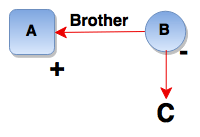# Blood Relations and Family Tree - Logical Reasoning (MCQ) Questions for Q. 29999

1. J + K means J is the son of K
2. J - K means J is the wife of K
3. J x K means J is the brother of K
4. J ÷ K means J is the mother of K
5. J = K means J is the sister of K

What does A x B ÷ C mean?

- Published on 15 May 17

a. A is the brother of C
b. A is the father of C
c. A is the uncle of C
d. A is the nephew of C

ANSWER: A is the uncle of C

A x B ÷ C means A is the brother of B who is the mother of C i.e. A is the uncle of C.## ➨ Post your comment / Share knowledgeEnter the code shown above:

(Note: If you cannot read the numbers in the above image, reload the page to generate a new one.)